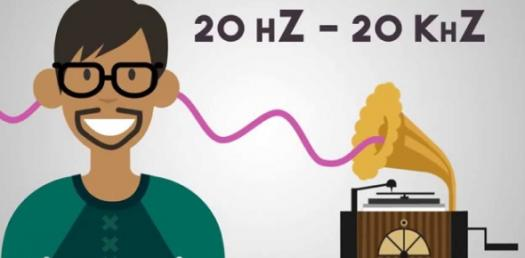# Physics Of Sound Post-test Troncoso

15 Questions | Total Attempts: 65SettingsThird grade FOSS physics of sound pretest

• 1.
Being able to tell different sounds apart is called
• A.

Sound amplification

• B.

Sound discrimination

• C.

Vibration

• D.

Frequency

• 2.
When you make sounds by plucking a rubber band, you make the rubber band move back and forth very quickly.  This movement is called
• A.

Loudness

• B.

Amplitude

• C.

Pitch

• D.

Vibration

• 3.
THe highness or lowness of a sound is the
• A.

Pitch

• B.

Loudness

• C.

Vibration

• D.

Resonance

• 4.
If you tighten a string and keep plucking it with your finger as you pull, what happens to the pitch?
• A.

As you tighten the string, the pitch gets lower

• B.

The pitch stays the same

• C.

As you tighten the string, the pitch gets higher

• D.

You can't tell unless you try it

• 5.
The loudness of a sound is called
• A.

The sound wave

• B.

The volume

• C.

The amplitude

• D.

The pitch

• 6.
A student holds two vibrating objects.  Object A is vibrating faster than object B.  Which statement is true?
• A.

A is louder than B

• B.

B is louder than A

• C.

A will have a higher pitch than B

• D.

B will have a higher pitch than A

• 7.
The same sound will seem louder if it travels through which of the following?
• A.

Solid objects

• B.

Liquids

• C.

Air

• D.

Solids, liquids and gases will sound the same

• 8.
If you roll a large sheet of paper into a funnel, you can hear the sound better.  This process of increasing the loudness of a sound is called
• A.

Measuring a wave

• B.

Absorption

• C.

Reflection

• D.

Amplification

• 9.
Which of the following would produce the hightest pitch?
• A.

Phone

• B.

Whistle

• C.

Truck horn

• D.

Dog's growl

• 10.
Which of the following would produce the loudest sound?
• A.

Jet plane

• B.

Pin falling

• C.

Doorbell

• D.

Whisper

• 11.
A unit for measuring the loudness of sounds is
• A.

Vibration

• B.

Decibel

• C.

Frequency

• D.

Pitch

• 12.
A process for locating distant or invisible objects by using sound waves that are reflected back to the sender
• A.

Vibration

• B.

• C.

Echolocation

• D.

Source

• 13.
The speed at which something vibrates is called
• A.

Pitch

• B.

Amplitude

• C.

Resonance

• D.

Frequency

• 14.
Something that detects sound is a
• A.

Sound wave

• B.

Volume

• C.

Amplitude

• D.

• 15.
An object or material that vibrates in a way that makes sound is called the
• A.

Sound source

• B.Back to top